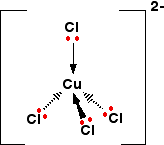# What would happen if "HCl" is added to a solution of cobalt (II) ions?

Apr 30, 2017

The green complex $\textsf{{\left[C u C {l}_{4}\right]}^{2 -}}$ is formed.

#### Explanation:

I am assuming we are in aqueous conditions.

The aqueous copper(II) ion consists of a central $\textsf{C {u}^{2 +}}$ ion surrounded by 6 $\textsf{{H}_{2} O}$ ligands:

It has the formula $\textsf{{\left[C u {\left({H}_{2} O\right)}_{6}\right]}^{2 +}}$ and is blue in colour. In solution it looks like this:If a large excess of chloride ions is added the water ligands are displaced as the following equilibrium is established:

$\textsf{{\left[C u {\left({H}_{2} O\right)}_{6}\right]}^{2 +} + 4 C {l}^{-} r i g h t \le f t h a r p \infty n s C u C {l}_{4}^{2 -} + 6 {H}_{2} O}$

stackrel(color(white)(xxxxxxxxxxxxxxxxxxxxxxxxxxxxxxxxxxxxx))(color(blue)(rarr)

$\textsf{\text{ "color(blue)(blue)" } \textcolor{g r e e n}{g r e e n}}$

Concentrated hydrochloric acid contains a large amount of chloride ions so Le Chatelier's Principle tells us that adding this will cause the position of equilibrium to shift to the right producing green $\textsf{C u C {l}_{4}^{2 -}}$ ions.

They have a tetrahedral structure:The solution looks like this:If excess water is now added the position of equilibrium is driven back to the left and the blue colour returns.

$\textsf{{\left[C u {\left({H}_{2} O\right)}_{6}\right]}^{2 +} + 4 C {l}^{-} r i g h t \le f t h a r p \infty n s C u C {l}_{4}^{2 -} + 6 {H}_{2} O}$

stackrel(color(white)(xxxxxxxxxxxxxxxxxxxxxxxxxxxxxxxxxxxxx))(color(blue)(larr)

$\textsf{\text{ "color(blue)(blue)" } \textcolor{g r e e n}{g r e e n}}$